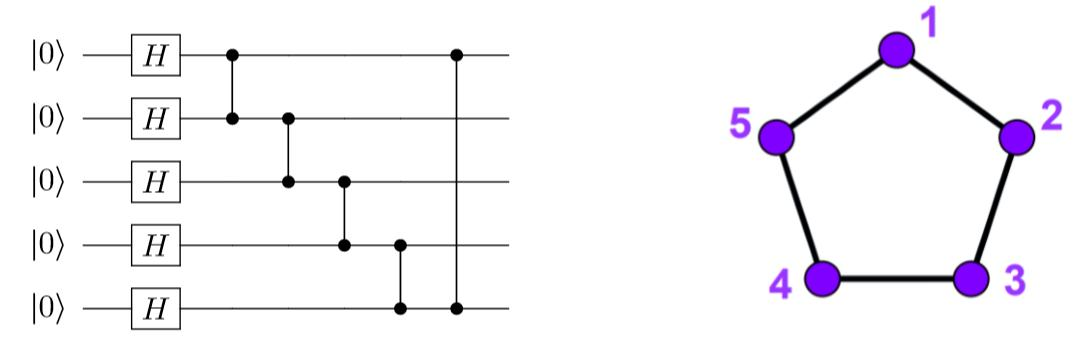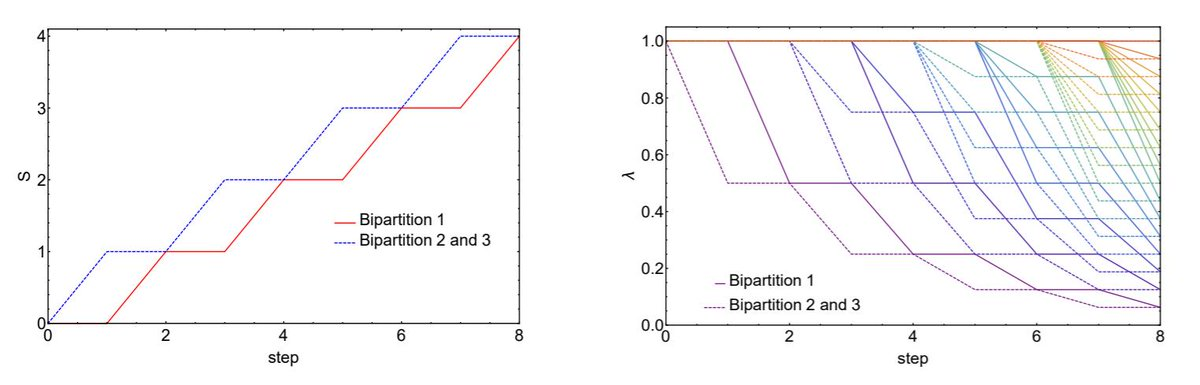# New article preprint: Quantum circuits for maximally entangled states

Last April 16, Alba Cervera-Lierta, José Ignacio Latorre and Dardo Goyeneche published an arXiv preprint titled “Quantum circuits for maximally entangled states”

One of the main goals of this article is to propose simple quantum circuits (short depth and basic gates) that can be used to test and compare current quantum computers.

Quantum computers should be able to generate and hold highly entangled states. Otherwise, we have very sophisticated classical techniques (such as tensor networks) that can simulate efficiently slightly entangled states.

Following this idea, they proposed to construct circuits that generate Absolutely Maximally Entangled (AME) states. AME states are those pure states which maximally entangle all their bipartitions. A simple way to construct an AME state is by using graph states, that is, states that can be constructed from a graph. Each graph vertex corresponds with the operation F|0> (F = Fourier gate) and each edge is a CZ gate. For example, this circuit generates the AME(5,2) (5 qudits of dimension 2, that is, 5 qubits). For qubits, the operation F|0> is just H|0>.The existence of AME states for any number of parties and any local dimension is an open problem. For more information, check Felix Huber table for a summary of known AME states:

For qubits, there only exist AME states for n=2 (Bell state), 3 (GHZ state), 5 and 6. This fact totally constraints the number of circuits that we can construct in current quantum computers… So they propose to “simulate” AME states of d>2 using qubits by implementing the mapping

|0> –> |00>,
|1> –> |01>,
|2> –> |10>,

With this mapping, one has “to adjust” the Fourier gates and the generalized CZ gates to multiqubit states. The explicit circuits and details about this mapping can be found in the main paper.

As a final remark, they also find an interesting property of these circuits. It turns out that AME (graph) state circuits majorize, that is, after applying each CZ gate (step), the entanglement of all bipartitions increases or remains equal, never decreases (entanglement measured with entropy S or eigenvalues of the reduced density matrix). In a sense, these circuits maximally entangle all their bipartitions in a very optimal way.Can we use this property to find and construct highly entangled states, new AME states or simplify current quantum circuits? We will see.

Finally, they implement an AME(5,2) state in a current quantum computer: 3 H gates and 5 CZ gates. The results are not very encouraging… But one should take into account that this is a very hard test for a quantum computer, to force it to maximally entangle all its parts!